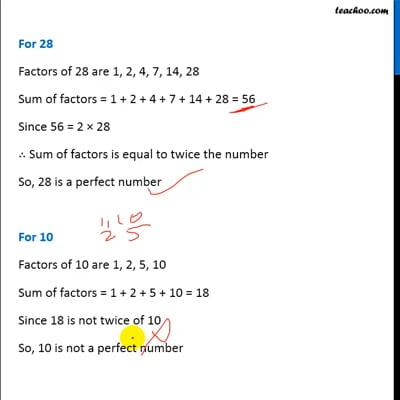Chapter 3 Class 6 Playing with Numbers
Concept wise

If Sum of all factors is equal to twice the number,

then number is called a perfect number

#### For 6

Factors of 6 are 1, 2, 3, 6

Sum of factors = 1 + 2 + 3 + 6 = 12

Since 12 = 2 × 6

∴ Sum of factors is equal to twice the number

So, 6 is a perfect number

#### For 28

Factors of 28 are 1, 2, 4, 7, 14, 28

Sum of factors = 1 + 2 + 4 + 7 + 14 + 28 = 56

Since 56 = 2 × 28

∴ Sum of factors is equal to twice the number

So, 28 is a perfect number

#### For 10

Factors of 10 are 1, 2, 5, 10

Sum of factors = 1 + 2 + 5 + 10 = 18

Since 18 is not twice of 10

So, 10 is not a perfect number

## First 10 perfect numbers are

6

28

496

8128

33550336

8589869056

137438691328

2305843008139952128

2658455991569831744654692615953842176

191561942608236107294793378084303638130997321548169216This video is only available for Teachoo black users